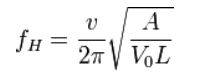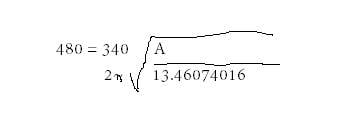# Helmholtz resonator equation. How do I do this? Please help.

Qaiphyx
Helmholtz resonator equation. How do I do this?? Please help.L = 0
Vo = 473.17 (I think its cubed though I calculated it from 16 ounces, so I should be cubed right??)
A = What im solving for
F = 480 hz
V= speed of sound which is 340.19 at sea level

If Vo needs to be cubed then A should be squared, but I dont know if Im supposed to do that

Ive put all this down on paper and im not sure how to run this equation to solve especially when it comes to the square root of a fraction, especially if the fraction is cubed and squared.

How do I do this??? Been searching the internet for 2 days now trying to figure this out.

## Answers and Replies

Homework Helper
Gold Member
Dearly Missed
What do you mean by cubed or squared??
You have a formula.
Solve it for A.

L cannot be 0, do you understand why?

Qaiphyx
Yes because then the it would be multiplied by Vo and would end in zero. By zero I me L is non existant in the equation, there is no neck to the helmholtz resonator. So L needs to be left out.

By cubed and squared I mean that it asked for Volume, the volume is 473.17 cubic centimeters. So it should be cubed right?? Really stupid question but I just want to make sure thats the case

Homework Helper
Gold Member
Dearly Missed
I'm sorry.
What you write is completely incomprehensible.
It doesn't seem that you even know what the number 0 is, nor what an equation is, either.

Qaiphyx
I'm sorry.
What you write is completely incomprehensible.
It doesn't seem that you even know what the number 0 is, nor what an equation is, either.

uh its very comprehensible.

Homework Helper
Gold Member
Dearly Missed
No, it is not
1. You can't put 0 in the denominator of an expression.
2. Volume cubed?? The volume stands there without any cubing done at all.
3. Why should you square A? You are to solve for it.

Qaiphyx
No, it is not
1. You can't put 0 in the denominator of an expression.
2. Volume cubed?? The volume stands there without any cubing done at all.
3. Why should you square A? You are to solve for it.

L is now .028448 centimeters. I guess you have to account for the thickness of the metal.

It what sense does the volume sit there without any cubing? Its 473.17 cubic centimeters. So, wouldnt you put in the equation as 473.17^3?

The result of A should be equal to centimeters squared correct? Thats just one other thing im unsure of. Is that I took the volume and converted it from ounces to cubic centimeters, so how should I input it in to the equation?

Homework Helper
Gold Member
Dearly Missed
"Its 473.17 cubic centimeters. So, wouldnt you put in the equation as 473.17^3?2
Why?
You have 473.17 cubic centimeters, not 473.17^3 cubic centimeters.

A cubic centimeter is a unit for volume counting.

Qaiphyx
"Its 473.17 cubic centimeters. So, wouldnt you put in the equation as 473.17^3?2
Why?
You have 473.17 cubic centimeters, not 473.17^3 cubic centimeters.

A cubic centimeter is a unit for volume counting.

Ok I understand then, that is what I was unsure of.

Let me try again to see if I can run this equation

Homework Helper
Gold Member
Dearly Missed
Run an equation?
Shouldn't you solve it for A??

QaiphyxThats where I got to, now what? and is it correct?

Qaiphyx
Run an equation?
Shouldn't you solve it for A??

thats what I meant by run it?

Homework Helper
Gold Member
Dearly Missed
Here's how you may do it:
1. NEVER insert numbers until your last step, solve it algebraically!
2. We have:
$$f_{H}=\frac{v}{2\pi}\sqrt{\frac{A}{V_{0}L}}$$
We multiply with 2pi/v, gaining:
$$\sqrt{\frac{A}{V_{0}L}}=\frac{2\pi{f}_{H}}{v}$$
We square both sides,
$$\frac{A}{V_{0}L}=\frac{4\pi^{2}{f}^{2}_{H}}{v^{2}}$$
We multiply with V_{0}L, and gain:
$$A=V_{0}L\frac{4\pi^{2}{f}^{2}_{H}}{v^{2}}$$
3. NOW, insert numbers to calculate A!

Mentor
3. NOW, insert numbers to calculate A!
If I may add: with units, otherwise you are in for a bad surprise.

Qaiphyx
Here's how you may do it:
1. NEVER insert numbers until your last step, solve it algebraically!
2. We have:
$$f_{H}=\frac{v}{2\pi}\sqrt{\frac{A}{V_{0}L}}$$
We multiply with 2pi/v, gaining:
$$\sqrt{\frac{A}{V_{0}L}}=\frac{2\pi{f}_{H}}{v}$$
We square both sides,
$$\frac{A}{V_{0}L}=\frac{4\pi^{2}{f}^{2}_{H}}{v^{2}}$$
We multiply with V_{0}L, and gain:
$$A=V_{0}L\frac{4\pi^{2}{f}^{2}_{H}}{v^{2}}$$
3. NOW, insert numbers to calculate A!

Thank you!

Qaiphyx
If I may add: with units, otherwise you are in for a bad surprise.

Har har har. Sorry im not a pro with math.

Mentor
If you just plug in the numbers, as you did in post #11, you will get the wrong answer because your units won't match.

Qaiphyx
Ok I did 240 hertz instead, so an octave lower, and got 1033.4 that looks a bit better.

Qaiphyx
So basically if what I have come up with is 9cm will give 120herts and 4.5 will give 60hertz.

Qaiphyx
Just to be sure, the speed of sound need to be converted to the same units that I am using for the resonator correct? In this case, centimeters